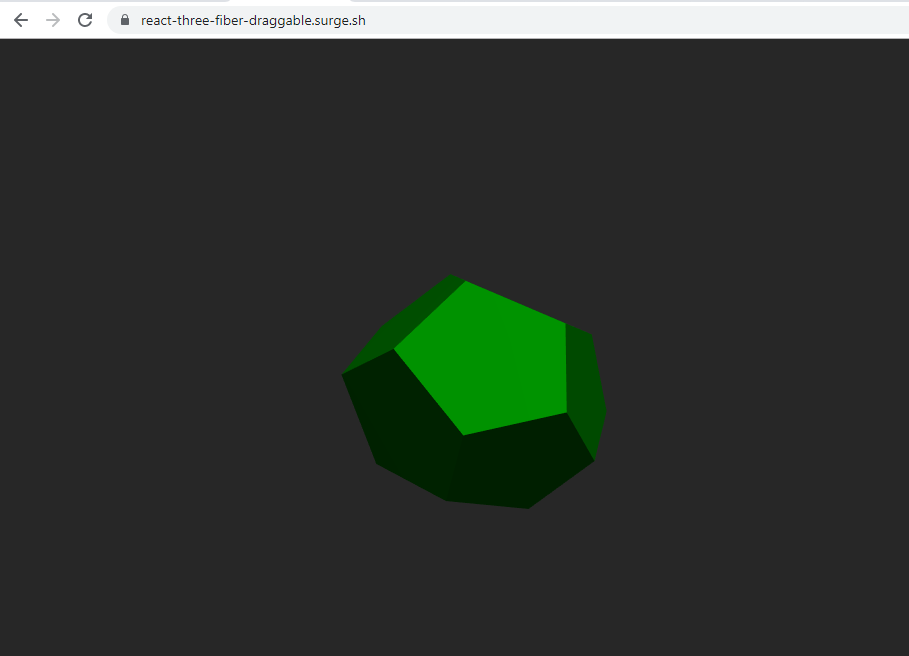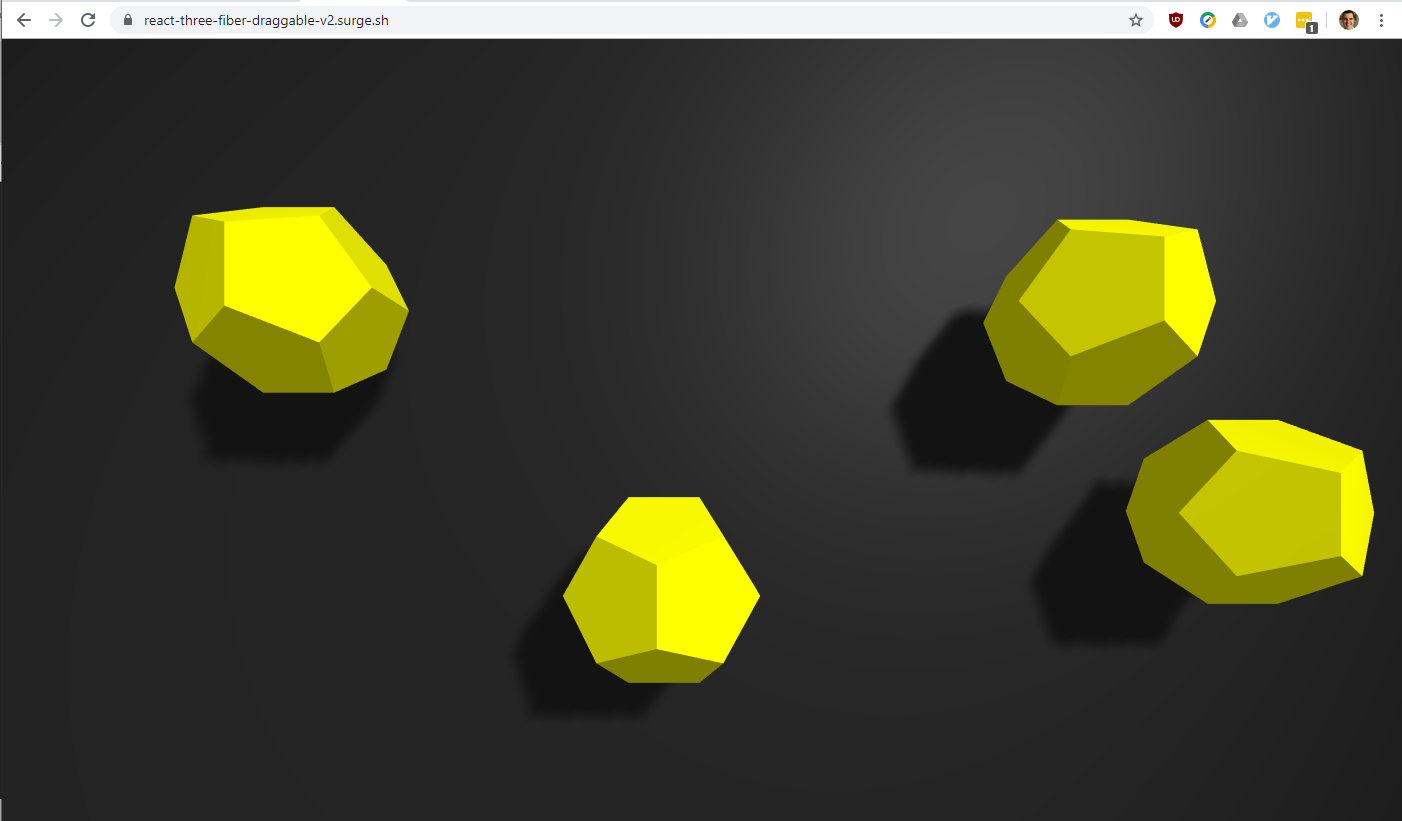# Create and Drag Shapes with Three.js, React and Cannon.js

by Max Rohde,

Following up from my article published a few days ago, I have now extended and improved the simple WebGL application that I originally developed using Three.js and react-three-fiber.

Version 1 of the application allowed dragging a simple shape around on the screen:Version 2 combines this basic premise with the cannon.js physics engine. Multiple objects can now be created and they drop down onto a solid plane, on which they can be moved.Simply click the canvas to add new shapes that then can be dragged around the plane.

The most important logic for this solution is in the `DraggableDodecahedron` component:

``````function DraggableDodecahedron({ position: initialPosition }) {
const { size, viewport } = useThree();
const [position, setPosition] = useState(initialPosition);
const [quaternion, setQuaternion] = useState([0, 0, 0, 0]);
const aspect = size.width / viewport.width;

const { ref, body } = useCannon(
{ bodyProps: { mass: 100000 } },
(body) => {
body.position.set(...position);
},
[]
);

const bind = useDrag(
({ offset: [,], xy: [x, y], first, last }) => {
if (first) {
body.mass = 0;
body.updateMassProperties();
} else if (last) {
body.mass = 10000;
body.updateMassProperties();
}
body.position.set(
(x - size.width / 2) / aspect,
-(y - size.height / 2) / aspect,
-0.7
);
},
{ pointerEvents: true }
);

useFrame(() => {
// Sync cannon body position with three js
const deltaX = Math.abs(body.position.x - position);
const deltaY = Math.abs(body.position.y - position);
const deltaZ = Math.abs(body.position.z - position);
if (deltaX > 0.001 || deltaY > 0.001 || deltaZ > 0.001) {
setPosition(body.position.clone().toArray());
}
const bodyQuaternion = body.quaternion.toArray();
const quaternionDelta = bodyQuaternion
.map((n, idx) => Math.abs(n - quaternion[idx]))
.reduce((acc, curr) => acc + curr);
if (quaternionDelta > 0.01) {
setQuaternion(body.quaternion.toArray());
}
});
return (
<mesh
ref={ref}
position={position}
quaternion={quaternion}
{...bind()}
onClick={(e) => {
e.stopPropagation();
}}
>
<dodecahedronBufferGeometry attach="geometry" />
<meshLambertMaterial attach="material" color="yellow" />
</mesh>
);
}
``````

Most notably here are three React hooks:

With the first hook we create a Cannon body that is set to the same dimension and position as the shape.

``````const { ref, body } = useCannon(
{ bodyProps: { mass: 100000 } },
(body) => {
body.position.set(...position);
},
[]
);
``````

In the second hook, we use react-use-gesture to react to drag events. We temporarily set the mass of the body/shape to be moved to 0 and reset it to the original mass once the drag gesture is complete. Finally we also set the position of the cannon.js body to the position that the drag gesture current indicates.

``````      const bind = useDrag(({ offset: [,], xy: [x, y], first, last }) => {
if (first) {
body.mass = 0;
body.updateMassProperties();
} else if (last) {
body.mass = 10000;
body.updateMassProperties();
}
body.position.set((x - size.width / 2) / aspect, -(y - size.height / 2) / aspect, -0.7);
}, { pointerEvents: true });
``````

The third hook, `useFrame()`, runs a callback function before every frame is rendered (this hook is supplied by react-three-fiber). This is used here to synchronise the position of the body in cannon.js with the three.js shape. Since cannon.js updates positions with a very fine granularity, we first assert that a body has changed its position or orientation by a significant margin. Only if this is the case, we update the shape. This helps React to avoid unnecessary updates of the 'virtual dom'.

``````    useFrame(() => {
// Sync cannon body position with three js
const deltaX = Math.abs(body.position.x - position);
const deltaY = Math.abs(body.position.y - position);
const deltaZ = Math.abs(body.position.z - position);
if (deltaX > 0.001 || deltaY > 0.001 || deltaZ > 0.001) {
setPosition(body.position.clone().toArray());
}
const bodyQuaternion = body.quaternion.toArray();
const quaternionDelta = bodyQuaternion.map((n, idx) => Math.abs(n - quaternion[idx]))
.reduce((acc, curr) => acc + curr);
if (quaternionDelta > 0.01) {
setQuaternion(body.quaternion.toArray());
}
});
``````

Apart from these hooks there is a simple click handler that stops click events from propagating. This is to prevent the event handler defined for the plane to trigger (this triggers adding a new shape to the scene).

Next I will be adding camera movement to this example.

Categories: javascript